# Obtuse Triangles

Go back to  'Triangles'

 1 Introduction to Triangle 2 Definition of Obtuse Triangle 3 Obtuse Angled Triangle Formula 4 Obtuse Angled Triangle Properties 5 Solved Examples 6 Tips and Tricks 7 Practice Questions 8 Important Notes 9 Maths Olympiad Sample Papers 10 Frequently Asked Questions(FAQs)

We at Cuemath believe that Math is a life skill. Our Math Experts focus on the “Why” behind the “What.” Students can explore from a huge range of interactive worksheets, visuals, simulations, practice tests, and more to understand a concept in depth.

Book a FREE trial class today! and experience Cuemath’s LIVE Online Class with your child.

## Introduction to Triangle

We know that triangles are 3-sided closed shapes made with 3 line segments.

Explore the different triangles and their elements visually using the simulation below.

## Definition of Obtuse Triangle

An obtuse triangle is a type of triangle where one of the vertex angles is greater than 90°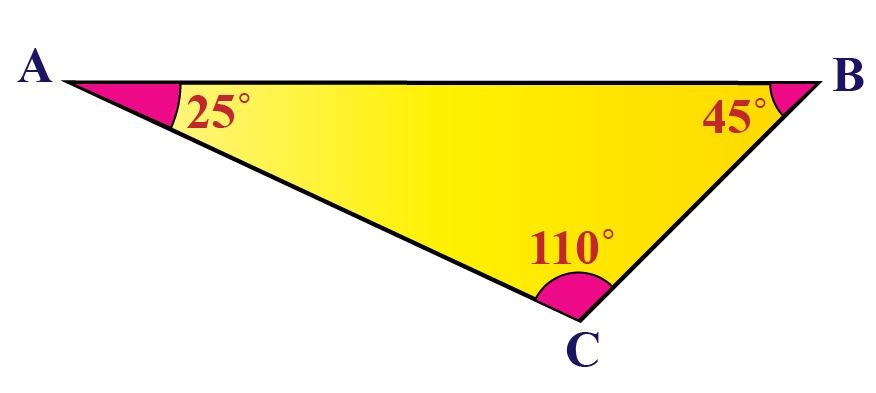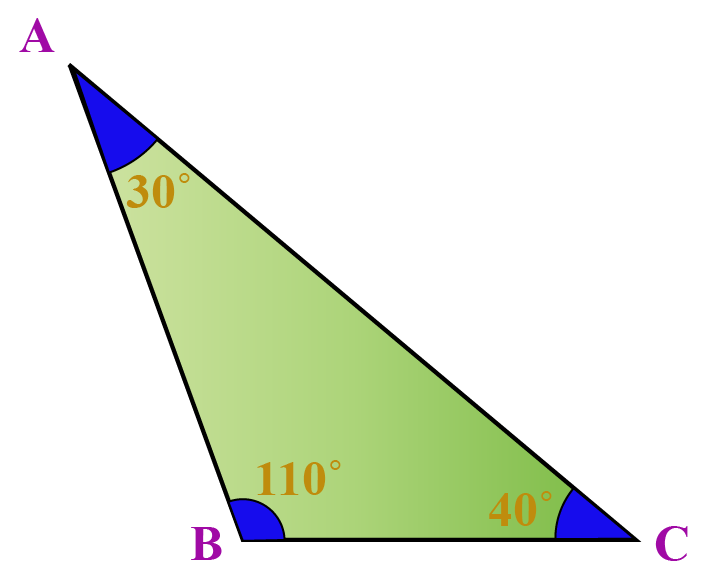The triangles above have one angle greater than 90°.

Hence, they are called obtuse-angled triangle or simply obtuse triangle.

An obtuse-angled triangle can be scalene or isosceles, but never equilateral.

## Obtuse Angled Triangle Formula

### Perimeter

The perimeter of an obtuse triangle is the sum of the measures of all its sides.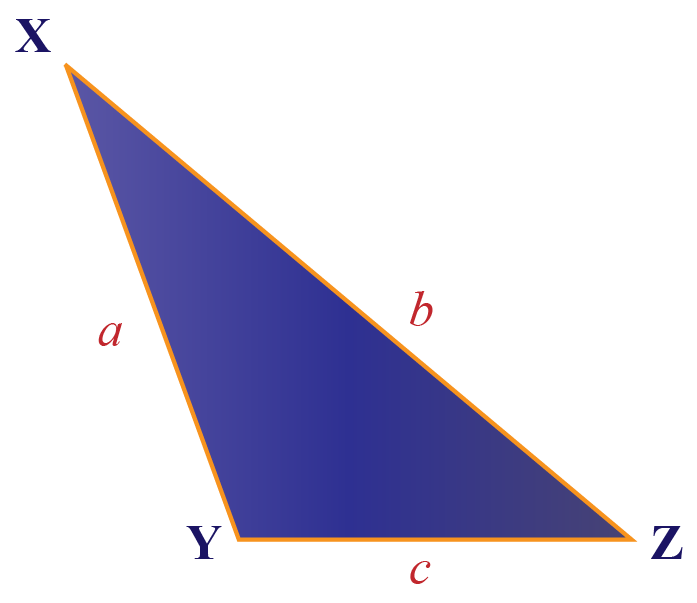Perimeter of obtuse triangle = a + b + c

### Area

In the given obtuse triangle $$ABC$$,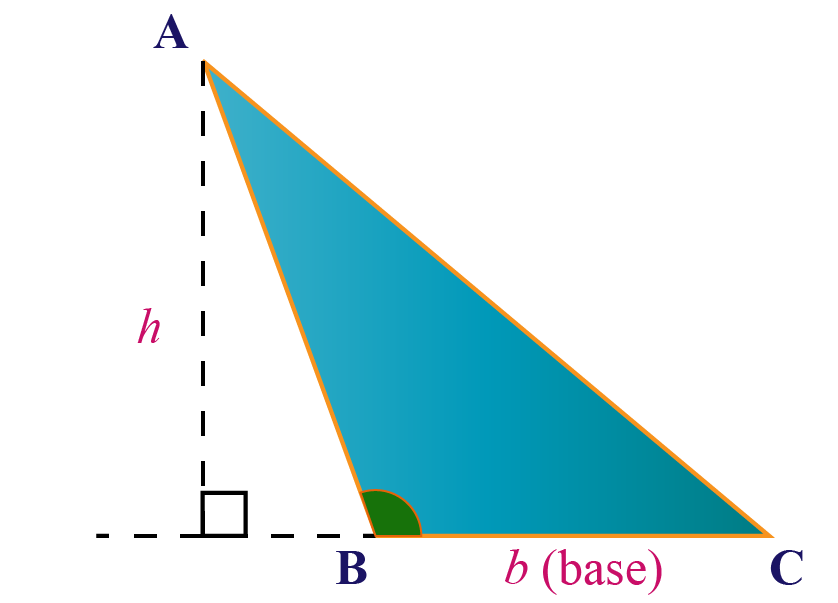\begin{align}\text{Area of }\Delta ABC = \frac{1}{2} h\times \text{b}\end{align}

$$\text{BC}$$ is the base and $$h$$ is the height of the triangle.

 Area = \begin{align}\frac{1}{2} \times \text{Base} \times \text{Height}\end{align}

We know that a triangle has 3 altitudes from the 3 vertices to the corresponding opposite sides.

The altitude or the height from the acute angles of an obtuse triangle lie outside the triangle.

We extend the base as shown and determine the height of the obtuse triangle.

We can also find the area of an obtuse triangle area using Heron's formula.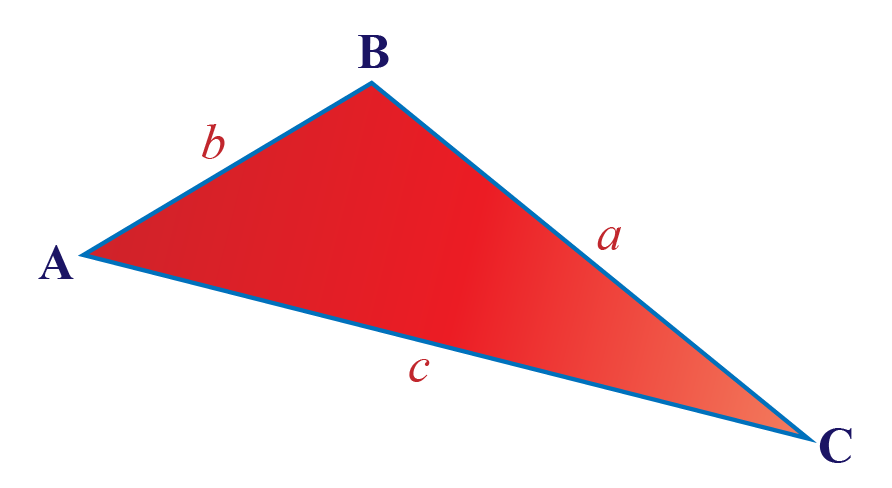Consider the triangle $$ABC$$ with sides $$a$$, $$b$$ and $$c$$.

Heron's formula to find the area of a triangle is:

 $$\sqrt {s(s - a)(s - b)(s - c)}$$

Note that $$(a + b + c)$$ is the perimeter of the triangle.

$$s$$ is the semi-perimeter which is given by:

$s = \frac{{a + b + c}}{2}$

## Obtuse Angled Triangle Properties

### Property 1

The longest side of a triangle is the side opposite to the obtuse angle.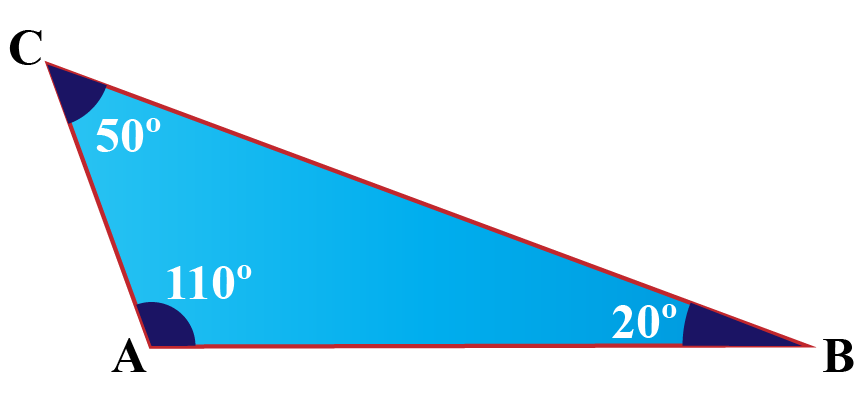The side BC is the longest side which is opposite to the obtuse angle $$\angle \text{A}$$

Check the proof of this property here.

### Property 2

A triangle cannot have more than one obtuse angle.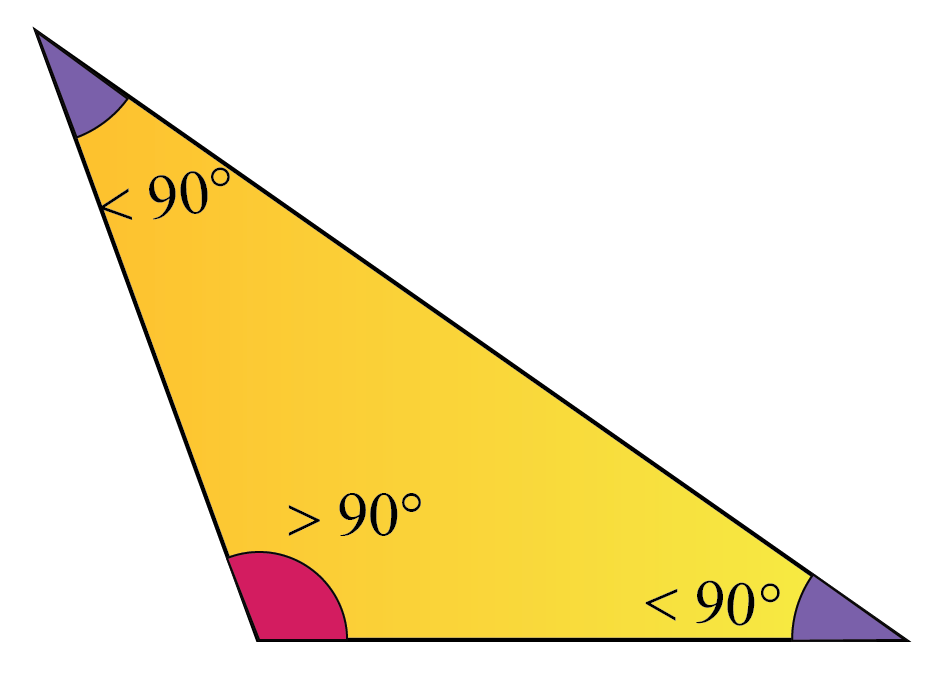We know that the angles of any triangle add up to 180°

Consider the obtuse triangle shown above.

We can observe that one of the angles measures greater than 90°, thus making it an obtuse angle.

Even if we assume this obtuse angle to be 91°, the other two angles of the triangle will add up to 89° degrees.

Hence, a triangle cannot have 2 obtuse angles.

### Property 3

Sum of the other two angles in an obtuse-angled triangle is less than 90$$^\circ$$

We just learnt that when one of the angles is an obtuse angle, the other two angles add up to less than 90°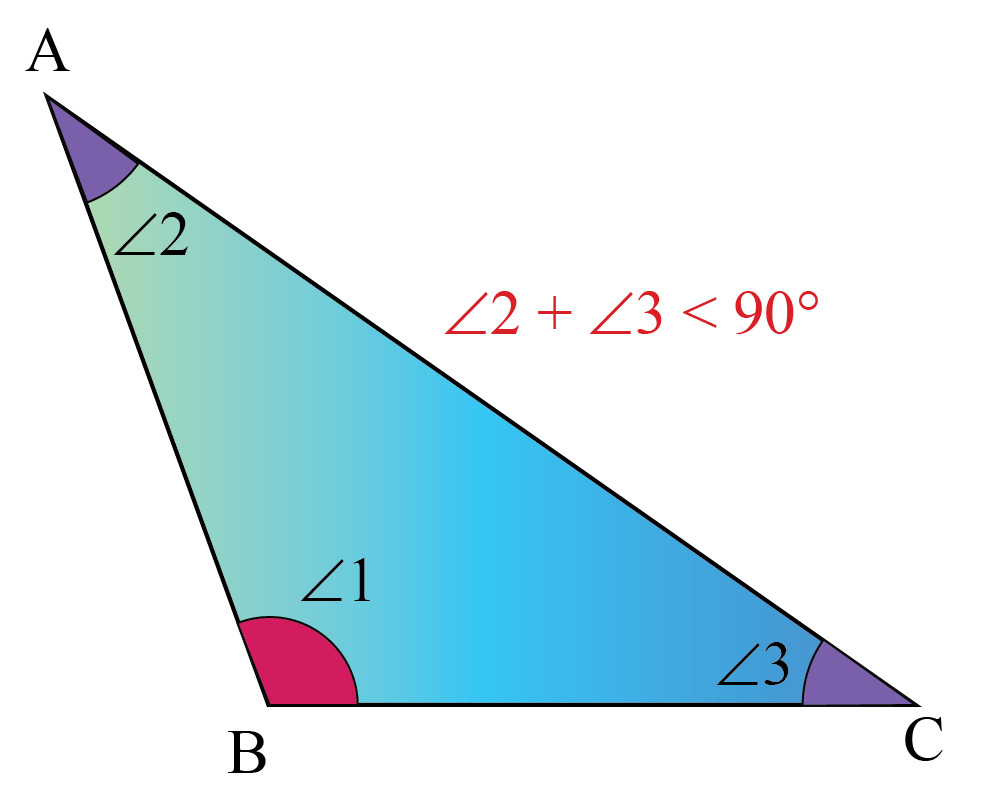In the above triangle,

$$\angle 1 >90^\circ$$

We know that by angle sum property, the sum of the angles of a triangle is 180°

Therefore,

$$\angle 1 + \angle 2 +\angle 3 = 180 ^\circ$$

and

$$\angle 1 >90^\circ$$

Subtracting the above two, we have:

$$\angle 2 +\angle 3 < 90 ^\circ$$

### Property 4

The circumcenter and the orthocenter of an obtuse-angled triangle lie outside the triangle.

The orthocenter (H), the point at which all the altitudes of a triangle intersect, lies outside in an obtuse triangle.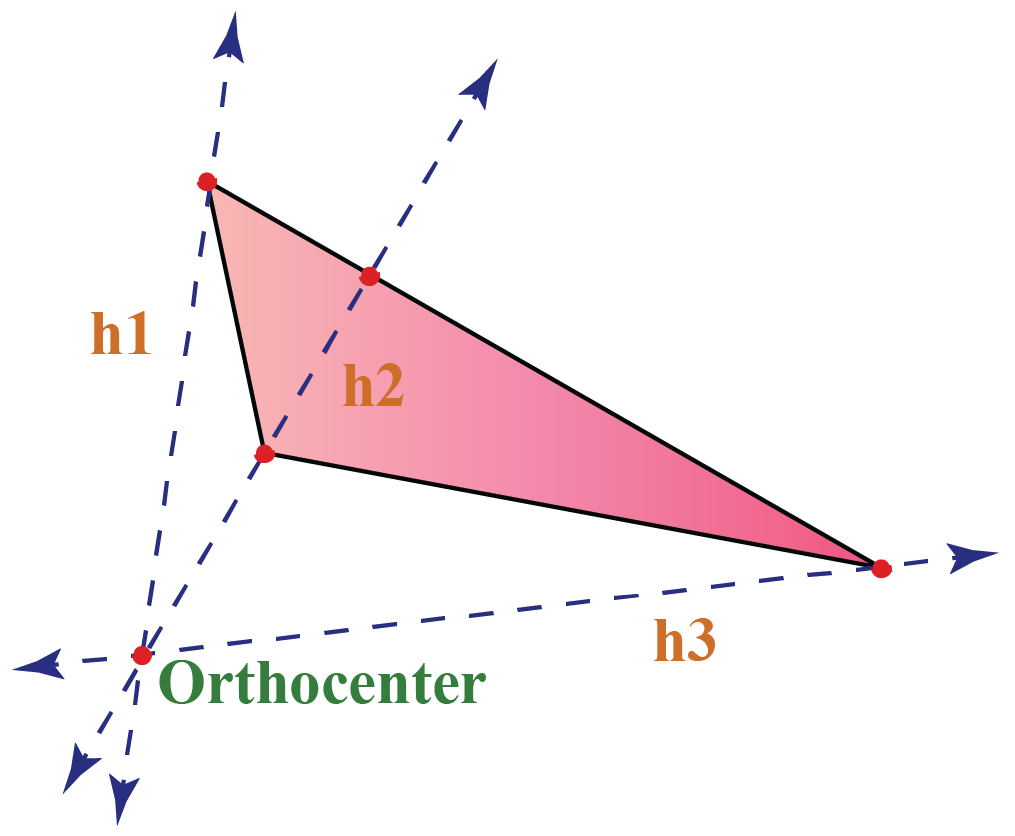Circumcenter (O), the point which is equidistant from all the vertices of a triangle, lies outside in an obtuse triangle.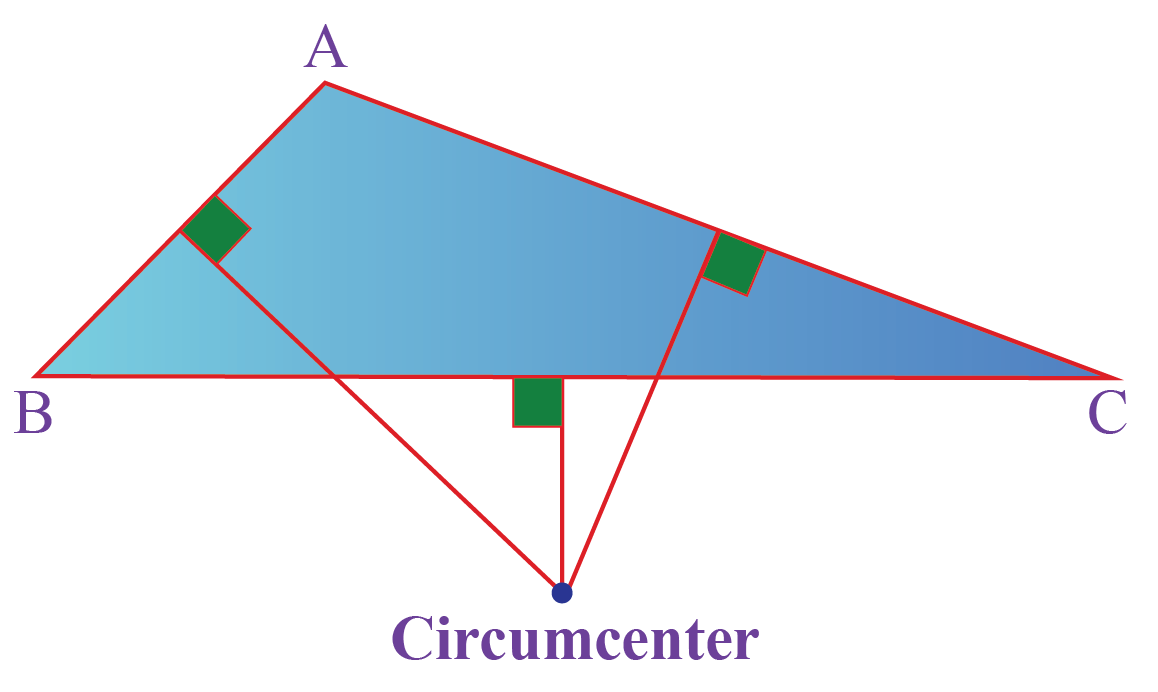Help your child score higher with Cuemath’s proprietary FREE Diagnostic Test. Get access to detailed reports, customised learning plans and a FREE counselling session. Attempt the test now.

## Solved Examples

 Example 1

Which of the following angle measures can form an obtuse triangle ABC?

a) 60°, 70°,50°

b) 95°, 30°, 55°

c) 89°,45°,46°

d) 90°, 60°, 30°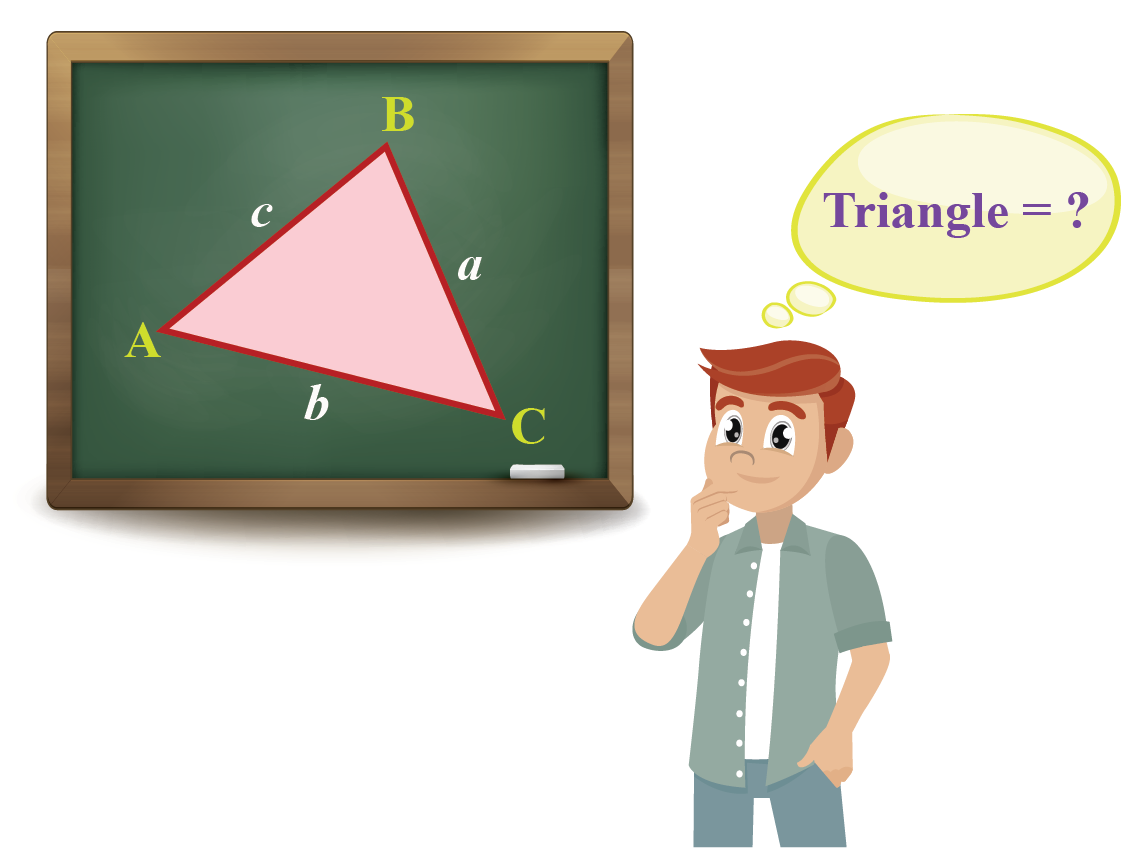Solution:

An obtuse triangle has one of the vertex angles as an obtuse angle (> 90°).

Among the given options, option (b) satisfies the condition.

 $$\therefore$$ Option b forms an obtuse triangle.
 Example 2

Find the area of an obtuse triangle whose base is 8 cm and height is 4 cm.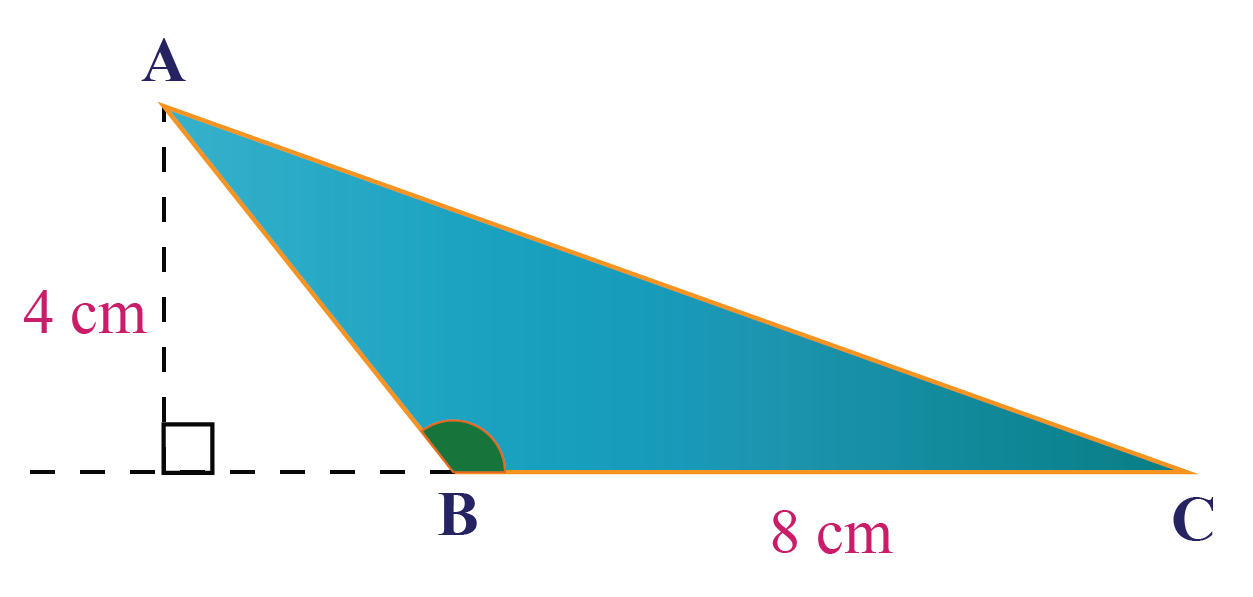Solution:

Area of an obtuse-angled triangle

= \begin{align}\frac{1}{2} \times \text{base} \times \text{height}\end{align}

Substituting the values of base and height, we get:

\begin {align} \text{Area} &= \frac{1}{2} \times 8 \times 4 \\ &= 16\: \text{cm}^2 \end{align}

 $$\therefore$$ Area of the triangle = 16 cm2
 Example 3

Find the height of the given obtuse triangle whose area = 60 cm2 and base = 8 cm.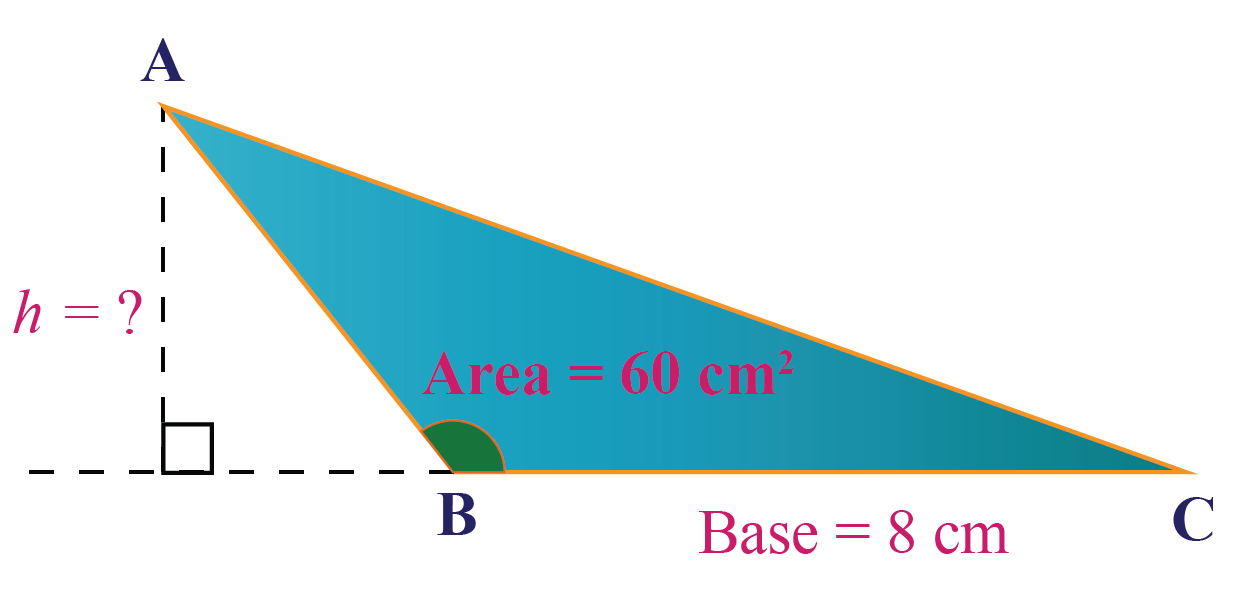Solution:

Area of an obtuse-angled triangle

= \begin{align}\frac{1}{2} \times \text{base} \times \text{height}\end{align}

Therefore, height of the obtuse triangle can be calculated by:

\begin{align}\text{Height} = \frac{2 \times \text{Area}}{\text{base}} \end{align}

Substituting the values, we get:

\begin {align} \text{Height} &= \frac{2 \times 60}{8 } \\ &= 15\: \text{cm} \end{align}

 $$\therefore$$ Height  = 15 cm
 Example 4

Can sides measuring 3 cm, 4 cm and 6 cm form an obtuse triangle?

Solution:

The sides of an obtuse triangle should satisfy the condition that the sum of the squares of any 2 sides is greater than the third side.

We know that

• $$a$$ = 3 cm
• $$b$$ = 4 cm
• $$c$$ = 6 cm

Taking the squares of the sides, we get:

$$a^2$$ = 9, $$b^2$$ = 16 and $$c^2$$ = 36

We know that

36 > (9 +16 )

Therefore, the given measures can form the sides of an obtuse triangle.

 3 cm, 4 cm and 6 cm can be the sides of an obtuse triangle.
 Example 5

A triangle with one exterior angle measuring 80° is shown in the image.

Can this triangle be an obtuse triangle?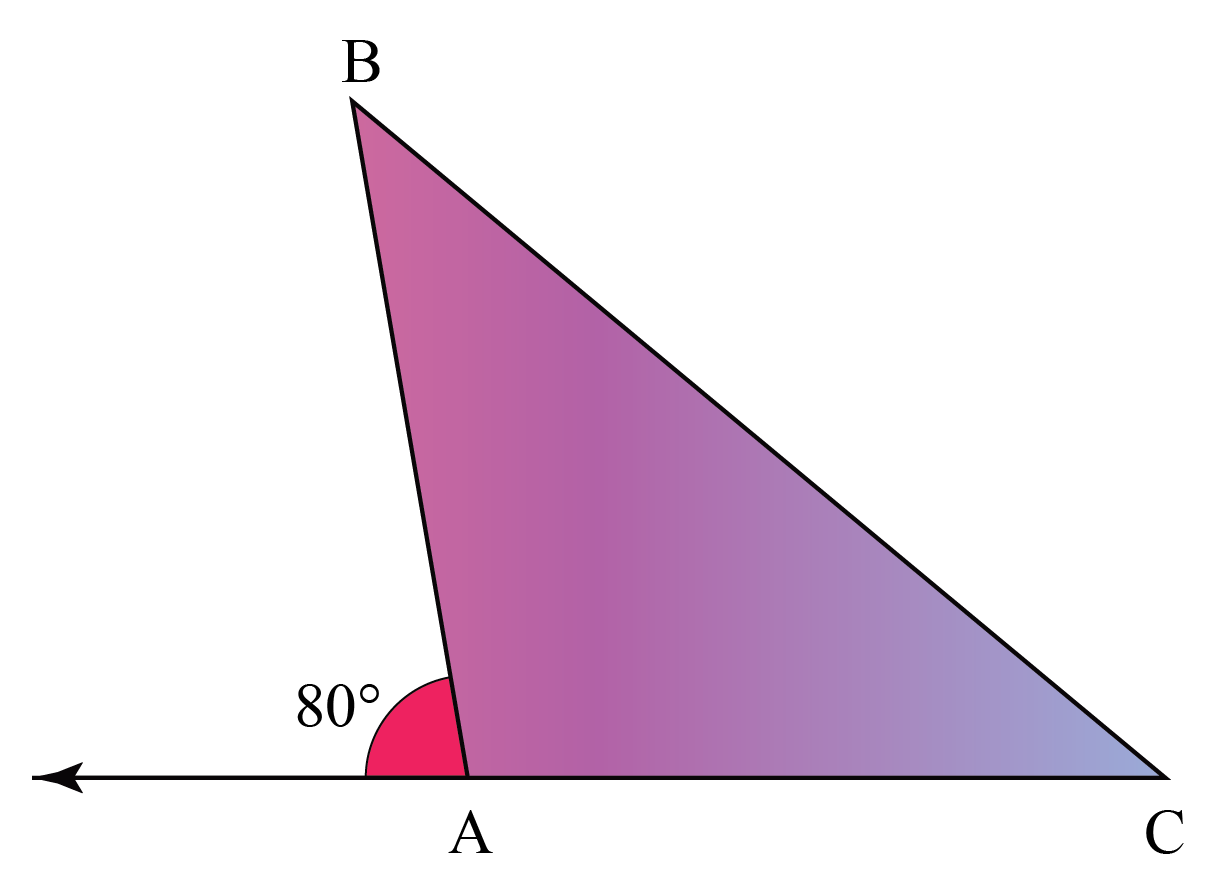Solution:

The exterior angle and the adjacent interior angle forms a linear pair (i.e , they add up to 180°).

Hence, the interior angle at vertex B is

180 - 80 = 100°

 $$\therefore$$ The given triangle is an obtuse-angled triangle.Tips and Tricks
1. If the 3 sides of a triangle measure $$a$$, $$b$$ and $$c$$ such that $$c$$ is the longest side of the triangle, the triangle is an obtuse-angled triangle if $$a^2 + b^2 < c^2$$
2. Centroid and incenter lie within the obtuse triangle while circumcenter and orthocenter lie outside the triangle.

Want to understand the “Why” behind the “What”? Explore  Obtuse Triangle with our Math Experts in Cuemath’s LIVE, Personalised and Interactive Online Classes.

Make your kid a Math Expert, Book a FREE trial class today!

## Practice Questions

Here are a few activities for you to practice.

IMO (International Maths Olympiad) is a competitive exam in Mathematics conducted annually for school students. It encourages children to develop their math solving skills from a competition perspective.

## 1. What is an obtuse triangle?

A triangle with one obtuse angle (greater than 90°) is called an obtuse triangle.

## 2. What is an example of an obtuse triangle?

A triangle where one angle is greater than 90° is an obtuse-angled triangle.

Example:  $$\Delta \text{ABC}$$ has these angle measures $$\angle \text{A} = 120^\circ , \angle \text{A} = 40^\circ , \angle \text{A} = 20^\circ$$

This triangle is an obtuse angled triangle because $$\angle \text{A} = 120^\circ$$

The following triangles are examples of obtuse triangles.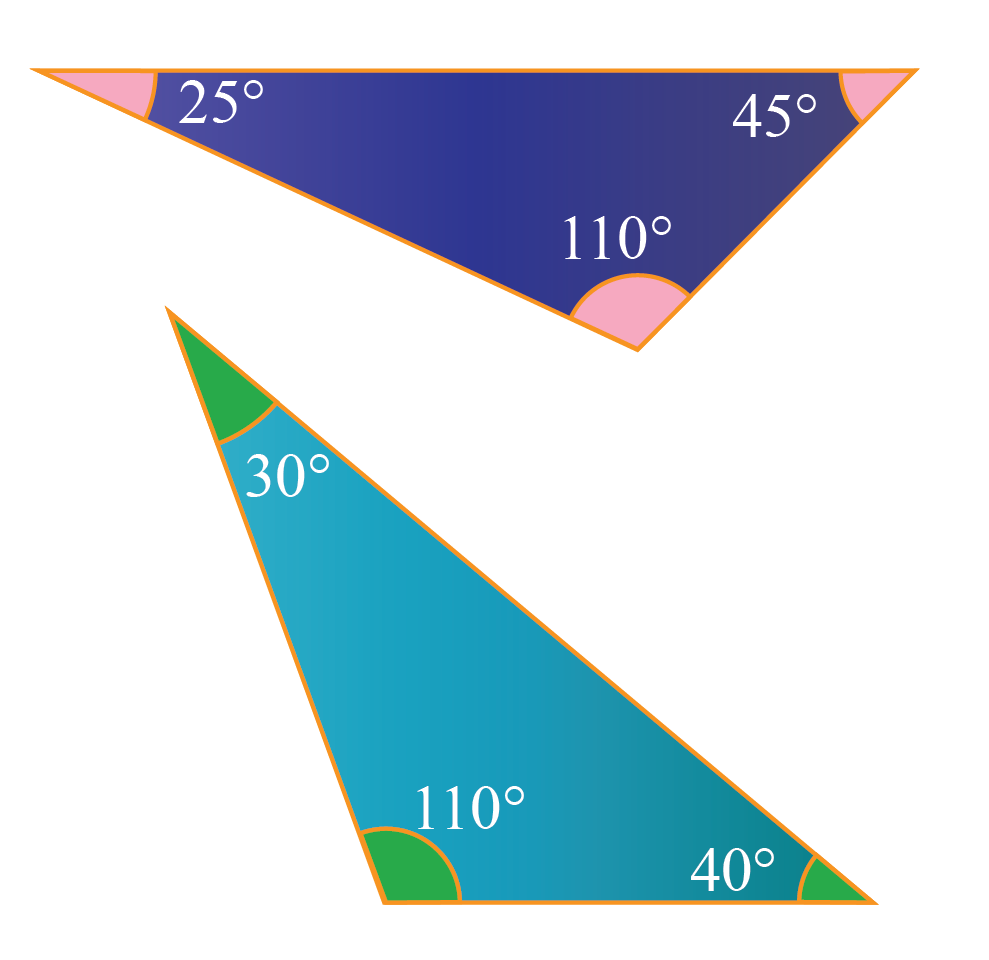## 3. What are the properties of obtuse triangles?

• The longest side of the triangle is the side opposite to the obtuse angle.
• A triangle cannot have more than one obtuse angle.
• Sum of the other two angles in an obtuse-angled triangle is less than 90$$^\circ$$.
• The circumcenter and the orthocenter of an obtuse-angled triangle lie outside the triangle.

More Important Topics
Numbers
Algebra
Geometry
Measurement
Money
Data
Trigonometry
Calculus